# Chapter 6.1. Nested Loops

In the current chapter, we will be looking at nested loops and how to use for loops to draw various figures on the console, that contain symbols and signs, ordered in rows and columns on the console. We will use single and nested loops (loops that stay in other loops), calculations, and checks, to print on the console simple and not so simple figures by specified sizes.

### Problem: Rectangle of 10 x 10 Stars

Print on the console a rectangle made out of 10 x 10 asterisks.

Input Output
(None) **********
**********
**********
**********
**********
**********
**********
**********
**********
**********

#### Hints and Guidelines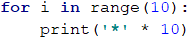How does the example work? We initialize a loop with a variable i. The default value of the variable is i = 0. With each iteration of the loop, the variable increases by 1 while it is less than 10. This way the code in the body of the loop is executed 10 times - from 0 to 9 included. In the body of the loop, we print a new line on the console '*' * 10, which creates a string of 10 asterisks.

### Problem: Rectangle of N x N Stars

Write a program that receives a positive number n and prints on the console a rectangle made out of N x N asterisks.

Input Output Input Output Input Output
2 **
**
3 ***
***
***
4 ****
****
****
****

#### Hints and Guidelines

The task is similar to the previous one:## Nested Loops

A nested loop is a construction where the body of one loop (the outer one) stays inside another loop (the inner one). In each iteration of the outer loop, the whole inner loop is executed. This happens in the following way:

• When nested loops start executing, the outer loop starts first: initialization of its control variable is performed and after checking for the end of the loop, the code in its body is executed.
• After that, the inner loop is executed. The control variable's start position is initialized, a check for the end of the loop is made and the code in its body is executed.
• When the set value for the end of the loop is reached, the program goes back one step up and continues executing the previous outer loop. The control variable of the outer loop changes with one step, a check is made to see if the condition for the end of the loop is met and a new execution of the nested (inner) loop is started.
• This is repeated until the variable of the outer loop meets the condition to end the loop.

Here is an example that illustrates nested loops. The aim is again to print a rectangle made of N x N asterisk, in which for each row a loop iterates from 1 to N, and for each column a nested loop is executed from 1 to N:In Python, when the standard initial value of the variable in the loop (i = 0) does not work for us, we can change it with the above syntax. I.e. when we want the loop to start from 1 and rotate to n inclusive, we write: for i in range (1, n + 1). The first value in parentheses indicates the beginning of the loop, and the second - the end of the loop, but not including, i.e. the loop ends before it is reached.

Let's look at the example above. After the initialization of the first (outer) loop, its body, which contains the second (nested) loop, starts to run. It prints one line n of asterisks. After the internal loop completes its execution in the first iteration of the external one, then the external one will continue, i.e. will print a blank line on the console. Then the first loop will be updated and the whole second (nested) loop will be executed again. The inner loop will be executed as many times as the body of the outer loop is executed, in this case, n** times.

### Problem: Square of Stars

Print on the console a square made of N x N asterisks:

Input Output Input Output Input Output
2 * *
* *
3 * * *
* * *
* * *
4 * * * *
* * * *
* * * *
* * * *

#### Hints and Guidelines

The problem is similar to the last one. The difference here is that we need to figure out how to add a whitespace after the asterisks so that there aren't any excess white spaces at the beginning and the end:### Problem: Triangle of Dollars

Write a program that receives an integer n and prints a triangle made of dollars of size n.

Input Output Input Output Input Output
3 $ $ $ $ 
4 $ $ $ $ 
 
5 $ $ $ $ 
 
  \$

#### Hints and Guidelines

The problem is similar to those for drawing a rectangle and square. Once again, we will use nested loops, but there is a catch here. The difference is that the number of columns that we need to print depends on the row, on which we are and not on the input number n. From the example input and output data, we see that the count of dollars depends on which row we are on at the moment of the printing, i.e. 1 dollar means the first row, 3 dollars mean the third row, and so on. Let's see the following example in detail. We see that the variable of the nested loop is connected with the variable of the outer one. This way our program prints the desired triangle:### Problem: Square Frame

Write a program that receives a positive integer n and draws on the console a square frame with a size of N x N.

Input Output Input Output
3 + - +
| - |
+ - +
4 + - - +
| - - |
| - - |
+ - - +
Input Output Input Output
5 + - - - +
| - - - |
| - - - |
| - - - |
+ - - - +
6 + - - - - +
| - - - - |
| - - - - |
| - - - - |
| - - - - |
+ - - - - +

#### Hints and Guidelines

We can solve the problem in the following way:

• We read from the console the number n.
• We print the upper part: first a + sign, then n-2 times - and in the end a + sign.
• We print the middle part: we print n-2 rows, as we first print a | sign, then n-2 times - and in the end again a | sign. We can do this with nested loops.
• We print the lower part: first a + sign, then n-2 times - and in the end a + sign.

Here is an example implementation of the above idea with nested loops:### Problem: Rhombus of Stars

Write a program that receives a positive integer n and prints a rhombus made of asterisks with size N.

Input Output Input Output
1 * 2  *
* *
 *
Input Output Input Output
3   *
 * *
* * *
 * *
  *
4    *
  * *
 * * *
* * * *
 * * *
  * *
   *

#### Hints and Guidelines

To solve this problem, we need to mentally divide the rhombus into two parts – the upper one, which also includes the middle row, and the lower one. For the printing of each part, we will use two separate loops, as we leave the reader to decide the dependency between n and the variables of the loops. For the first loop we can use the following guidelines:

• We print n-row whitespaces.
• We print *.
• We print row-1 times *.

The second (lower) part will be printed similarly, which again we leave to the reader to do.In Python, the standard step of the for loop is positive and is equal to 1. If we want to change it, we must use a third parameter in the arguments of the loop: for i in range (0, 100, 2). The third parameter, in this case, shows that the variable will increase from 0 to 99 inclusive, with step 2.

### Problem: Christmas Tree

Write a program that receives a number n (1 ≤ n ≤ 100) and prints a Christmas tree with a height of N + 1.

Input Output Input Output
1   |
* | *
2    |
 * | *
** | **
Input Output Input Output
3     |
  * | *
 ** | **
*** | ***
4      |
   * | *
  ** | **
 *** | ***
**** | ****

#### Hints and Guidelines

From the examples, we see that the Christmas tree can be divided into three logical parts. The first part is the asterisks and the whitespaces before and after them, the middle part is |, and the last part is again asterisks, but this time there are whitespaces only before them. The printing can be done with only one loop and the operation string multiplication, which we will use once for the asterisks and once for the whitespaces:## Drawing More Complex Figures

Let's look at how to draw figures using nested loops with more complex logic, for which we need to think more before coding.

### Problem: Sunglasses

Write a program that receives an integer n (3 ≤ n ≤ 100) and prints sunglasses with a size of 5*N x N as found in the examples:

Input Output Input Output
3 ******   ******
*////*|||*////*
******   ******
4 ********    ********
*//////*||||*//////*
*//////*    *//////*
********    ********
Input Output
5 **********     **********
*////////*     *////////*
*////////*|||||*////////*
*////////*     *////////*
**********     **********

#### Hints and Guidelines

From the examples, we can see that the sunglasses can be divided into three parts – upper, middle, and lower. Below is part of the code with which the problem can be solved. When drawing the upper and lower rows we need to print 2 * n asterisks, n whitespaces, and 2 * n asterisks:When drawing the middle part, we need to check if the row is (n-1) / 2 - 1 because in the examples we can see that in this row we need to print vertical slashes instead of whitespaces: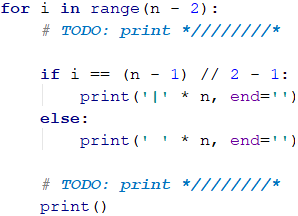### Problem: House

Write a program that receives a number n (2 ≤ n ≤ 100) and prints a house with size N x N, just as in the examples:

Input Output Input Output Input Output
2 **
||
3 -*-
***
|*|
4 -**-
****
|**|
|**|
Input Output Input Output
5 --*--
-***-
*****
|***|
|***|
8 ---**---
--****--
-******-
********
|******|
|******|
|******|
|******|

#### Hints and Guidelines

We understand from the description of the problem that the house is with a size of n x n. What we see from the example input and output is that:

• The house is divided into two parts: roof and base.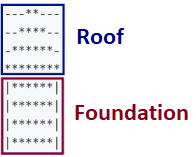• When n is an even number, the point of the house is "dull".
• When n is odd, the roof has a sharp point and is one row larger than the base.
##### The Roof
• It comprises asterisks and dashes.
• At its highest part, there are one or two asterisks, depending on whether n is even or odd, as well as dashes.
• At its lowest part, there are many asterisks and little to no dashes.
• With each lower row, the asterisks increase by 2, and the dashes decrease also by 2.
##### The Base
• The height is n rows.
• It is made out of asterisks and vertical slashes.
• Each row comprises 2 vertical slashes – one in the beginning and one at the end of the row, and also asterisks between the vertical slashes with a string length of n - 2.

We read the input number n from the console and write its value in a variable: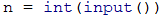It is very important to check if the input data is correct! In these tasks, it is not a problem to directly convert the data from the console into type int, because it is said that we will be given valid integers. If you are making more complex programs, it is a good practice to check the data. What will happen if instead of a number the user inputs the character "A"?

To draw the roof, we write down how many asterisks we start with a variable called stars:

• If n is an odd number, there will be 1 star.
• If it is even, there will be 2.Calculate the length of the roof. It equals half of n. Write the result in the variable roof_length: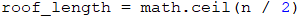Note: To use math.ceil (), which rounds to the larger integer, regardless of the fractional part, it is necessary to import the library math. This is done with the command import math. It is recommended to write import math (as well as all other imports) at the beginning of the file.

It is important to note that when n is an odd number, the length of the roof is one row more than that of the base.

In Python, when two integer types are divisible and there is a remainder, the result will be a number with a remainder. If we want to perform a pure integer division without a remainder, it is necessary to use the operator //.

Example:

result1 = 3 / 2    # result 1.5
result2 = 3 // 2   # result 1


If we want to round up to the next largest integer number, we need to use the method math.cail(…): result = math.ceil(3 / 2).

After we have calculated the length of the roof, we make a loop from 0 to roof_length. On each iteration we will:

• Calculate the number of dashes we need to draw. The number will be equal to (n - stars) / 2. We store it in variable padding.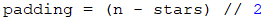• We print on the console: "dashes" (padding times) + "asterisks" (stars times) + "dashes" (padding times):• Before the iteration is over, we add 2 to stars (the number of the asterisks).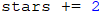After we have finished with the roof, it is time for the base. It is easier to print:

• We start with a loop from 0 to n (exclusive).
• We print on the console: | + * (n - 2 times) + |.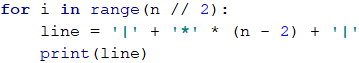If we have written everything correctly, our problem should be solved.

### Problem: Diamond

Write a program that receives an integer n (1 ≤ n ≤ 100) and prints a diamond with size N, as in the following examples:

Input Output Input Output Input Output
1 *
2 ** 3 -*-
*-*
-*-
Input Output Input Output Input Output
4 -**-
*--*
-**-
5 --*--
-*-*-
*---*
-*-*-
--*--
6 --**--
-*--*-
*----*
-*--*-
--**--
Input Output Input Output Input Output
7 ---*---
--*-*--
-*---*-
*-----*
-*---*-
--*-*--
---*---
8 ---**---
--*--*--
-*----*-
*------*
-*----*-
--*--*--
---**---
9 ----*----
---*-*---
--*---*--
-*-----*-
*-------*
-*-----*-
--*---*--
---*-*---
----*----

#### Hints and Guidelines

What we know from the problem's description is that the diamond is n x n in size. From the example input and output we can conclude that all rows contain exactly n symbols, and all the rows, except for the top and bottom ones, have 2 asterisks. We can mentally divide the diamond into 2 parts:

• Upper part. It starts from the upper tip down to the middle.
• Lower part. It starts from the row below the middle one and goes down to the lower tip (inclusive).
##### Upper Part
• If n is an odd number, it starts with 1 asterisk.
• If n is an even number, it starts with 2 asterisks.
• With each row down, the asterisks get further away from each other.
• The space between, before, and after the asterisks is filled up with dashes.
##### Lower Part
• With each row down, the asterisks get closer to each other. This means that space (the dashes) between them is getting smaller and space (the dashes) on the left and the right is getting larger.
• The bottom-most part has 1 or 2 asterisks, depending on whether n is an even or odd number.
##### Upper and Lower Parts of The Diamond
• On each row, except the middle one, the asterisks are surrounded by inner and outer dashes.
• On each row, there is space between the two asterisks, except on the first and the last row (sometimes the asterisk is 1).

We read the value of n from the console, converting it to type int: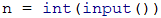We start drawing the upper part of the diamond. The first thing we need to do is to calculate the number of the outer dashes left_right (the dashes on the outer side of the asterisks). It is equal to (n - 1) / 2, rounded down:After we have calculated left_right, we start drawing the upper part of the diamond. We can start by running a loop from (n + 1) // 2 (i.e. rounded down).

At each iteration of the loop the following steps must be taken:

• We print on the console the left dashes (with length left_right) and right after them the first asterisk: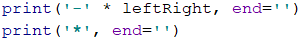• We will calculate the distance between the two asterisks. We can do this by subtracting from n the number of the outer dashes, and the number 2 (the number of the asterisks, i.e. the diamond's outline). We need to store the result of the subtraction in a variable mid.• If the mid is lower than 0, we know that on the row there should be only 1 star. If it is higher or equal to 0 then we have to print dashes with length mid and one asterisk after them.

• We print on the console the right outer dashes with length left_right.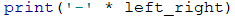• At the end of the loop, we decrease left_right by 1 (the asterisks are moving away from each other).

We are ready with the upper part.

Printing the lower part is very similar to that of the upper part. The difference is that instead of decreasing left_right with 1 at the end of the loop, we will increase it with 1 at the beginning of the loop. Also, the loop will iterate from 0 to (n - 1) // 2: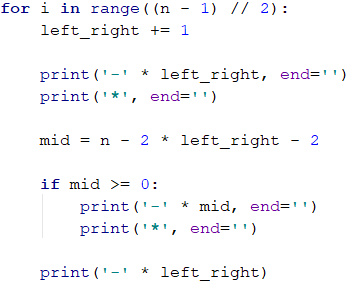Repeating a code is considered bad practice because the code becomes very hard to maintain. Let's imagine that we have a piece of code (e.g. the logic for drawing a row from the diamond) in a few more places and we decide to change it. To do this, we will have to go through all the places and change it everywhere. Now let's imagine that you need to reuse a piece of code not 1, 2, or 3 times but tens of times. A way to overcome this problem is to use functions. You can search for more information about them on the Internet or view Chapter “10” (Functions). </tr></table>

If we have written all correctly, then the problem is solved.

## What Have We Learned from This Chapter?

We learned about one of the ways to create strings:

print_me = '*' * 5


We learned how to draw figures using nested for loops:

for row in range(5):
print('*', end='')

for col in range(4):
print(' *', end='')

print()


## Lab: Drawing Figures in a Web Environment

Now that we got used to the nested loops and the way to use them to draw figures on the console, we can get into something even more interesting: we can see how loops can be used to draw in a Web environment. We will make a web application that visualizes a number rating (a number from 0 to 100) with stars. This kind of visualization is common in e-commerce sites, reviews of products, event rating, rating of apps, and others.

Don't worry if you don't understand all of the code, how exactly it is written and how the project works. It is normal, now we are learning to write code and we are a long way from the web development technologies. If you are struggling to write your project by following the steps, ask for help in the SoftUni's Reddit Community: https://www.reddit.com/r/softuni/.

### Problem: Ratings – Visualization in a Web Environment

Develop a web application for rating visualization (number from 0 to 100). Draw from 1 to 10 stars (with halves). Stars to be generated with a for cycle.#### Hints and Guidelines

We start by creating a new project in PyCharm from [File] -> [New Project] (or from the start window):We give a meaningful name to the project, for example "Ratings". We choose the type of the current Python interpreter. Let this be the default: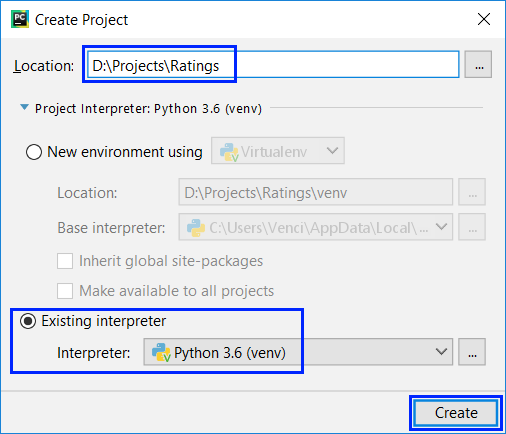We will again use the Flask library, which is used to create web applications. Before we can start coding, we need to install Flask. Let's recall how to do this. We go to the settings of PyCharm [File] -> [Settings] -> [Project: Ratings] -> [Project Interpreter]. There, we press the + button: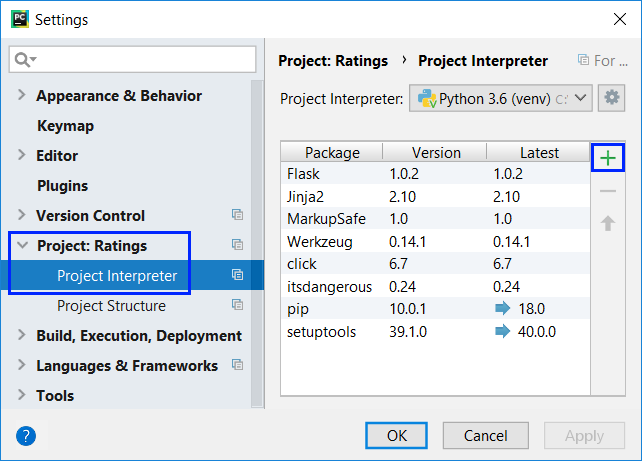Look for Flask in the window that appears and click the [Install Package] button: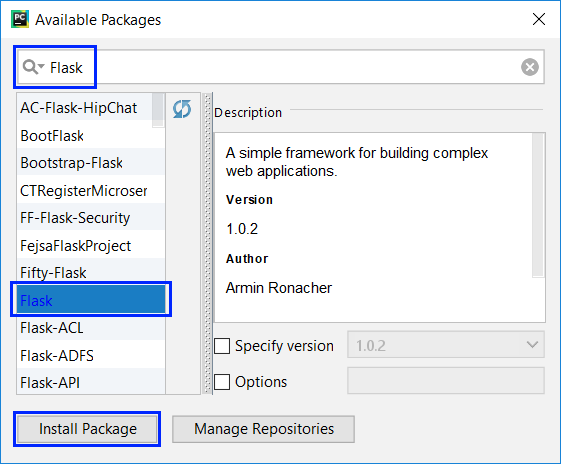We are now adding the structure of the project (the assignment files for this project can be downloaded from here). Copy them from Windows Explorer and paste them in the Ratings project folder with Copy/Paste: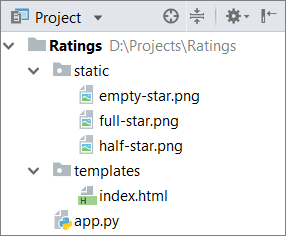For everything to work, we need to add the code. First, we go to the file index.html (from the templates folder) and look for the TODO sections. In their place we enter the following code: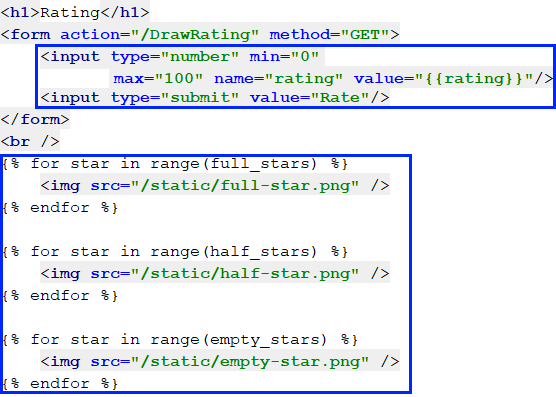The above code creates a web form <form> with one field " rating " for entering a number in the interval [0… 100] and a button [Rate] to send the data from the form to the server. Then, draw with three separate for loops the corresponding number of stars - filled, half-empty and empty.

The action that will process the data is called /DrawRatings, which means the function draw_ratings () in the file app.py: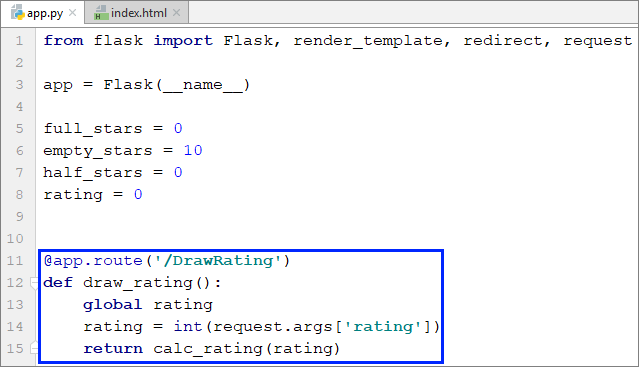The code from the function draw_ratings () takes the entered number rating from the form and passes it to the function calc_rating (…). The calc_rating (…) function computes and calculates the number of full stars, the number of empty stars, and the number of halves of stars, then reload the page, but with new ones submitted values of the variables for the stars. We implement it as follows: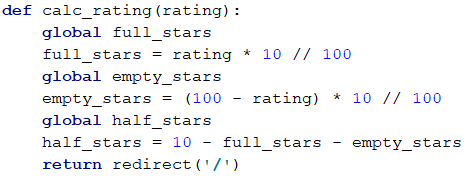We start the project with [Ctrl+Shift+F10] (or with [Right button] -> [Run 'app']) and wait for it to load: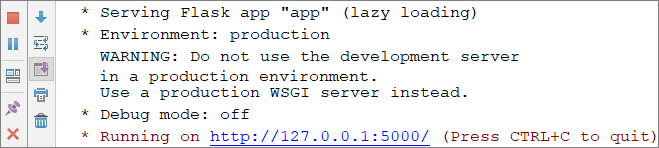We go to the specified address and enjoy the finished project:If you have problems with the sample project above, you can ask questions in the SoftUni's Reddit Community: https://www.reddit.com/r/softuni/.